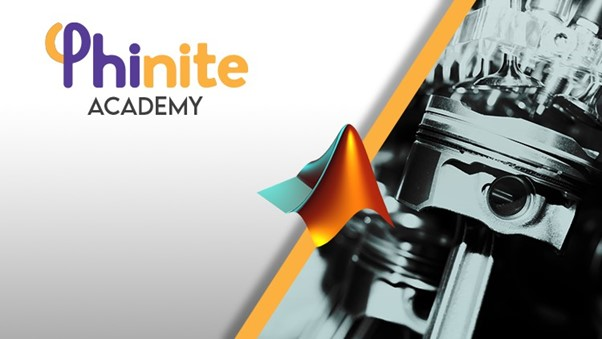# Crankshaft Modelling with System Dynamics

You'll learn how to model a Crankshaft in Matlab by using System Dynamics methods!

Language - English Published on 10/2021

• Crank-Shaft Model Introduction
04:43
Preview
• Free Body Diagram of Crank-Shaft Design
13:08
Preview
• System Parameters - Spring Factor
09:45
• Dynamic Equation for Crank with Spring and Damping Factors
11:48
• State-Space Form of Crank Model
06:22
• Correction Form of Crank Model
05:37
• MATLAB Modelling Application -1
19:15
• MATLAB Modelling Application -2
13:45

#### Description

In this course, how to create system dynamics models, which are one of the basic areas of mechatronic systems and which are actively used in mathematical modeling applications, are explained. The use of analogy models and their relationship with energy principles in an active form is given in this course.

The problem of transforming theory into practice or transferring the theory to a simulation environment, which is one of the biggest problems of engineering students, will be tried to be overcome with MATLAB applications. The SIMULINK interface has been actively preferred in the Matlab application. This program is aimed to model the systems in real-time/iterative and to get time responses. In addition, the input values are given to the system and the effect of these values on the result is discussed.

In order to realize the system modeling, especially the equations of motion have been tried to be implemented by Transfer Function and State-Space Model. The main purpose of the system modeling applications given on the frequency base is to obtain the characteristics of linear systems and to create an interface for the development of tools to be used in control problems. In the State-Space model, it is shown how the system can be modeled even in a nonlinear state. The active use of "Matlab Function" expressions in the MATLAB/SIMULINK program especially for Script Application is one of the contents of this course.

In addition, as another problem, how the system parameters that need to be transferred to real-life are equated in theory and turned into design parameters is one of the active outputs of this course. It is aimed to clarify the designs in a time-dependent model, especially with the understanding of mass/spring/damper analogies and obtaining the material selection/design parameters in 3-Dimensional space.

I hope our lesson will be useful for you :)

#### What Will I Get ?

• Mechatronics and System Dynamics

• Transfer Function and State Space Representation

• Control Theory

• Crank - Shaft System

• Vehicle Dynamics

• Vehicle Wheels

#### Requirements

• Basic Math Knowledge

• Basic Matlab Knowledge

#### Feedbacks (0)

• No Feedbacks Posted Yet..!This Course Includes :

1 hours

8 Lectures